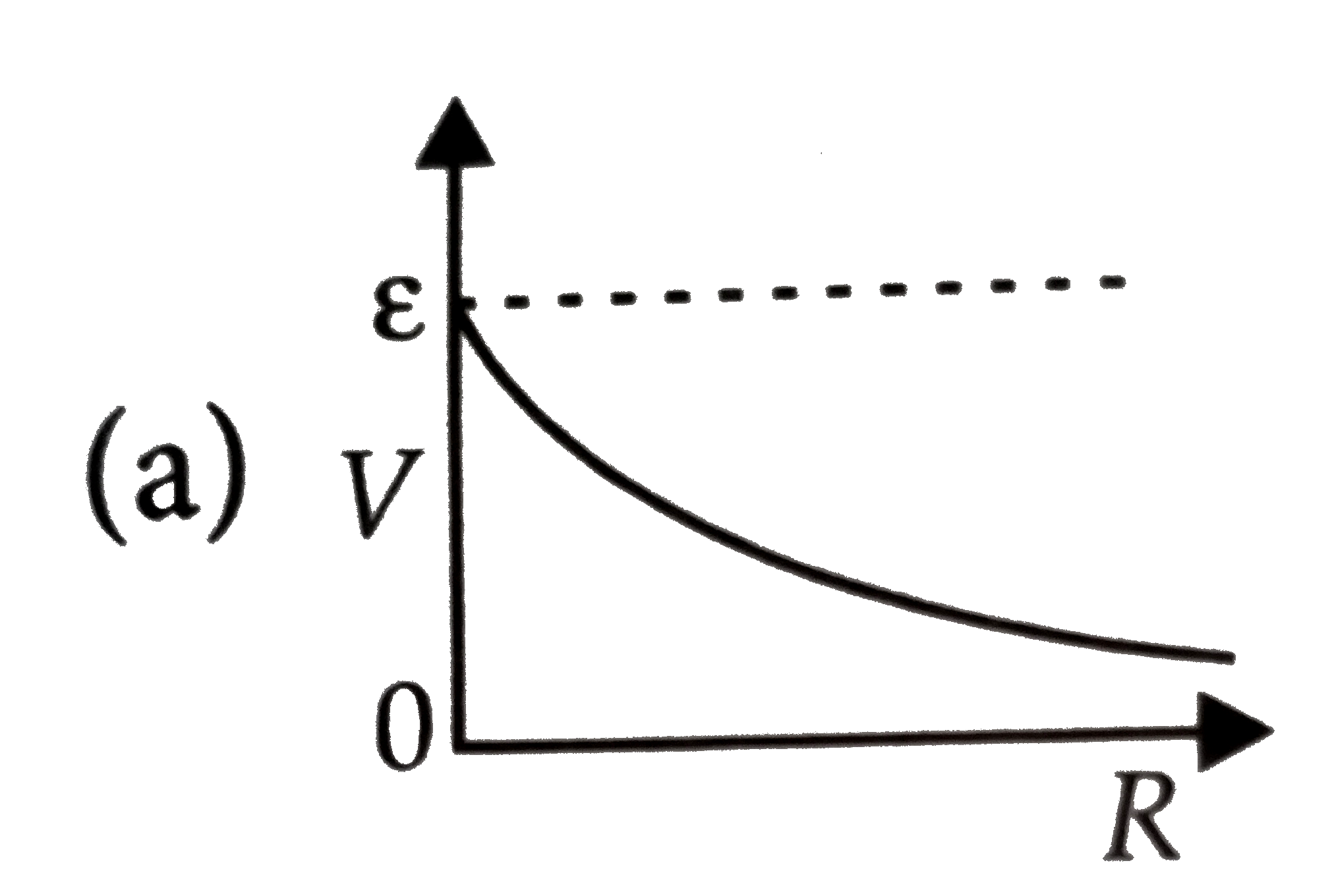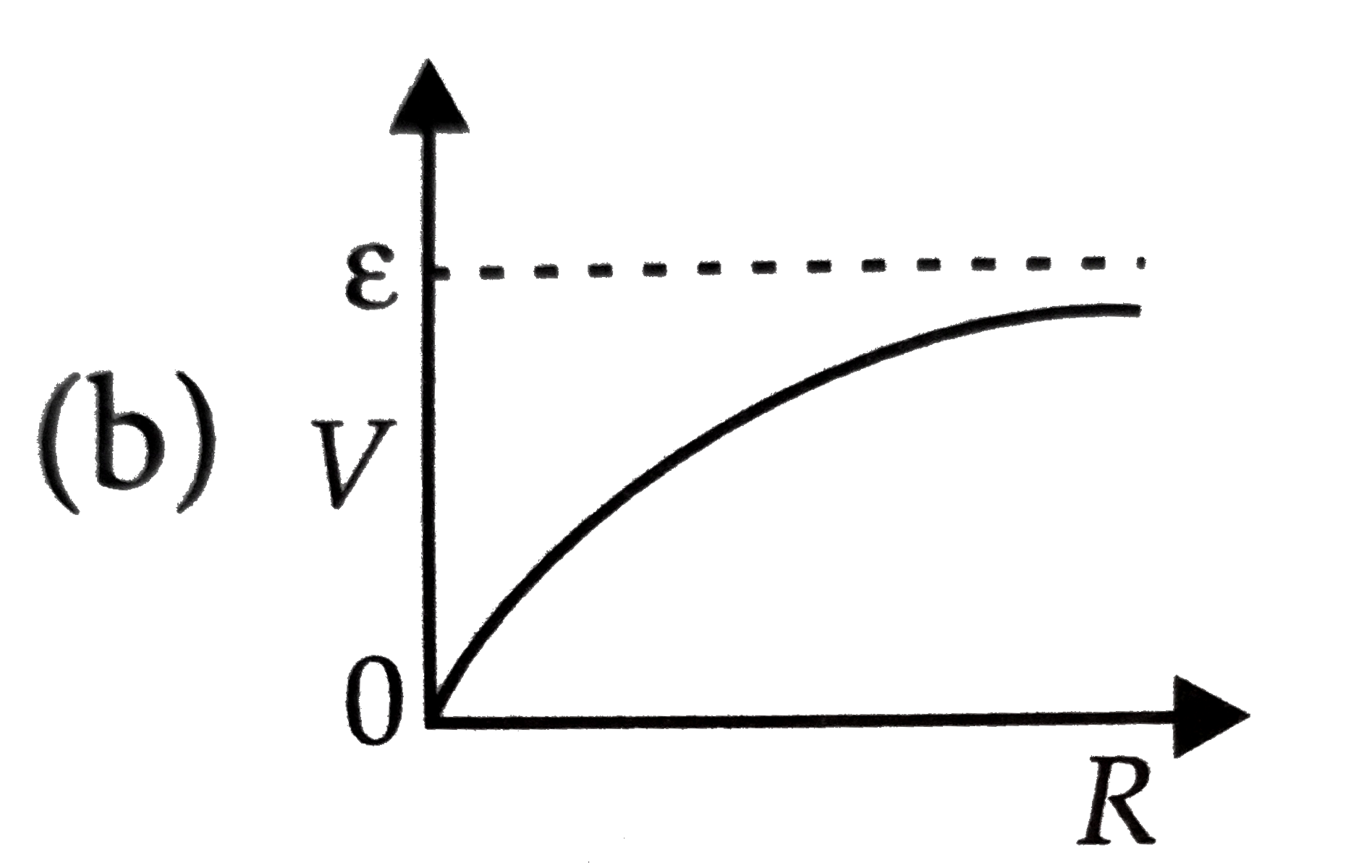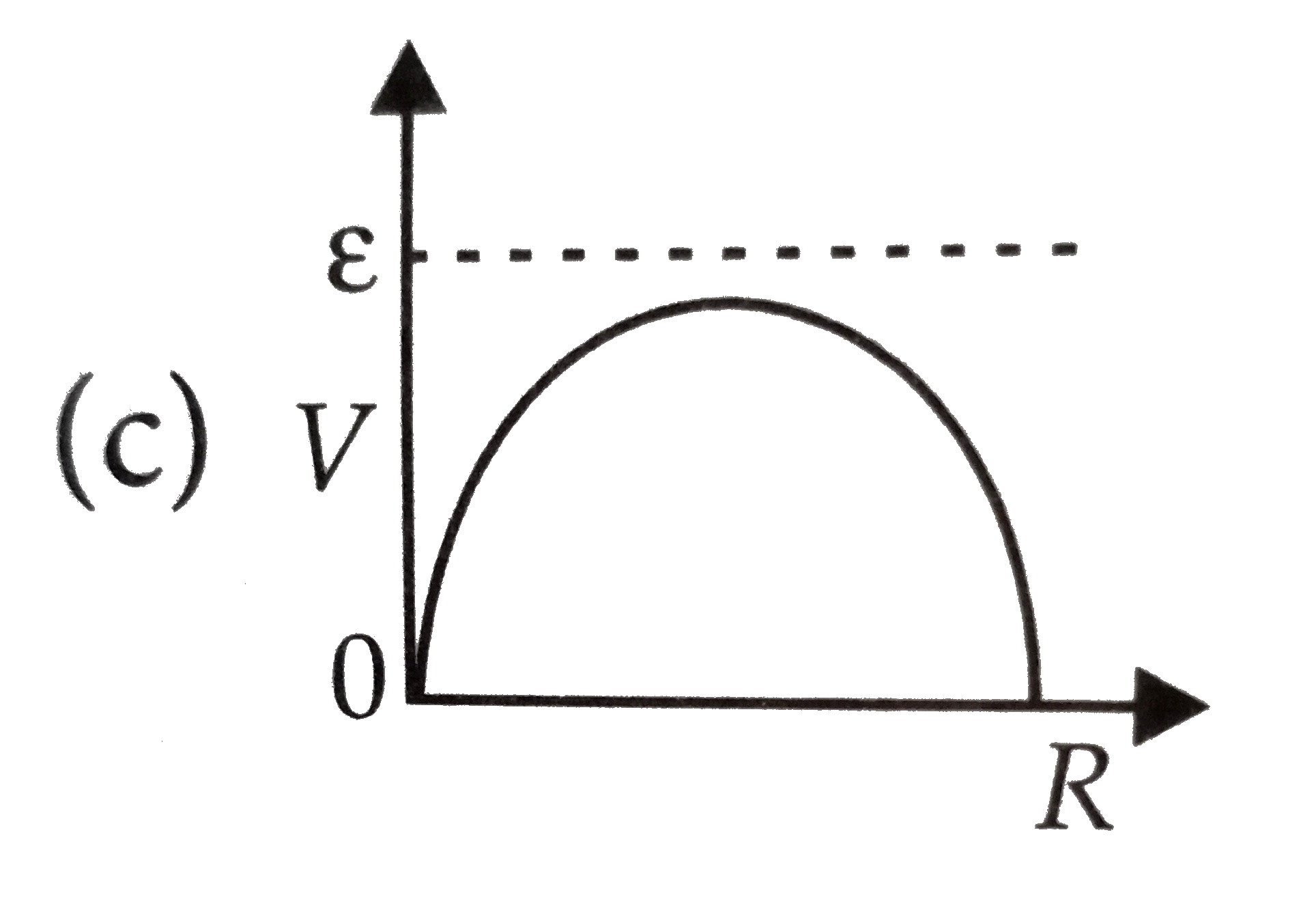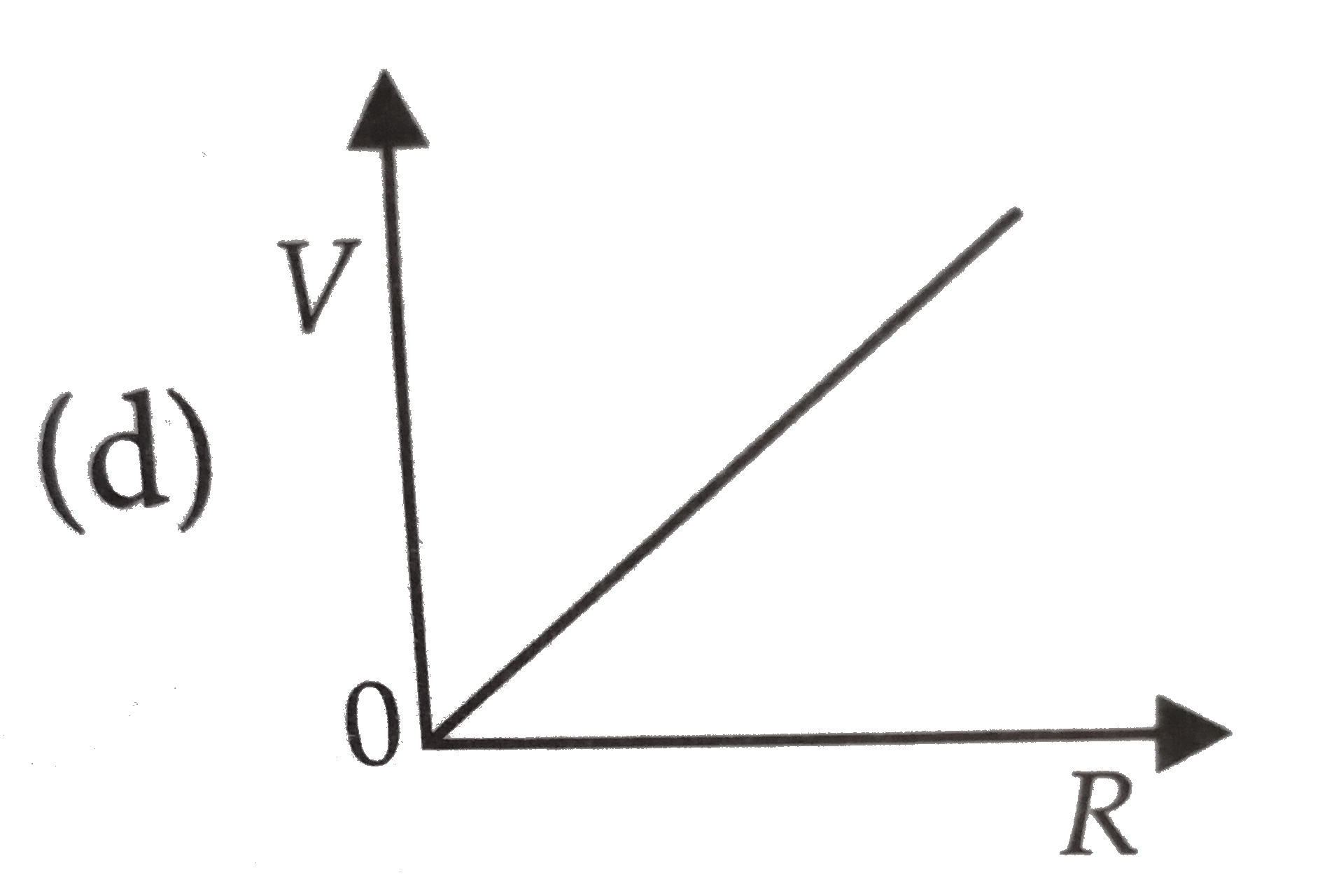# A cell having an emf E and internal resistance r is connected across a variable external resistance R. As the resistance R is increased, the plot of potential difference V across R is given byKhareedo DN Pro and dekho sari videos bina kisi ad ki rukaavat ke!

##### Updated On: 27-06-2022

लिखित उत्तर

ABCDSolution
Current in the circuit I=εR+r
Potential difference across R,
V=IR=(εR+r)=ε(1+rR)
When R=0,V=0orR=,V=εउत्तर

Step by step solution by experts to help you in doubt clearance & scoring excellent marks in exams.

Transcript

having a real and internal resistance R is connected across a variable acceleration resistance R is the resistance R is increased the flop of is connected to the substitute 10 asking you to find out the relevant Graham Ankur options how is the potential difference between two or more about how to potential difference varies with resistance when we are sure to find used to know about how we and our related for now the current is always given which is equal to bi R + are the capital as an external resistance and smaller as an internal resistance R is equal
to from in this will substitute for it so that will get it be divided 5r plus our into our shooting on the value sorry can justify out with one more step you can write V is equal to be provided by this are divided fore bottle ticket to the denominator are by Rs 1 + RBI now in this variation of privacy have if you give me as a zero then capital are will also be equal to zero if you give as infinity-infinity the denominator denominators denominator coaster
numerator and so we becomes equal to Fir becomes infinity is equal to what you are you have seen these conditions = 0 is equal to zero it is true in this is equal to zero is equal to zero all the three conditions of the first became involved then is equal to b is equal to 8 infinite value it should read a very large value if this is an infinite value at that infinite value do whatever the wheat is getting this matching with AC is equal to option V is the right answer

संबंधित वीडियो